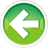# 5.3 — Modulus and Exponentiation

The modulus operator

The modulus operator (also informally known as the remainder operator) is an operator that returns the remainder after doing an integer division. For example, 7 / 4 = 1 remainder 3. Therefore, 7 % 4 = 3. As another example, 25 / 7 = 3 remainder 4, thus 25 % 7 = 4. Modulus only works with integer operands.

Modulus is most useful for testing whether a number is evenly divisible by another number: if x % y evaluates to 0, then we know that x is evenly divisible by y.

Here are a couple runs of this program:

```Enter an integer: 6
Enter another integer: 3
The remainder is: 0
6 is divisible by 3
```
```Enter an integer: 6
Enter another integer: 4
The remainder is: 2
6 is not divisible by 4
```

Modulus with negative numbers

The modulus operator can also work with negative operands. As of C++11, `x % y` always returns results with the sign of x.

Running the above program:

```Enter an integer: -6
Enter another integer: 4
The remainder is: -2
-6 is not divisible by 4
```
```Enter an integer: 6
Enter another integer: -4
The remainder is: 2
6 is not divisible by -4
```

In both cases, you can see the remainder takes the sign of the first operand.

Warning

Prior to C++11, modulus with a negative operand could result in either a positive or negative result. This was made predictable in C++11.

Where’s the exponent operator?

You’ll note that the ^ operator (commonly used to denote exponentiation in mathematics) is a Bitwise XOR operation in C++ (covered in lesson %Failed lesson reference, id XX%). C++ does not include an exponent operator.

To do exponents in C++, #include the <cmath> header, and use the pow() function:

Note that the parameters (and return value) of function pow() are of type double. Due to rounding errors in floating point numbers, the results of pow() may not be precise (even if you pass it integers or whole numbers).

If you want to do integer exponentiation, you’re best off using your own function to do so. The following function implements integer exponentiation (using the non-intuitive “exponentiation by squaring” algorithm for efficiency):

Don’t worry if you don’t understand how this function works -- you don’t need to understand it in order to call it.

Produces:

```13841287201
```

Warning

In the vast majority of cases, integer exponentiation will overflow the integral type. This is likely why such a function wasn’t included in the standard library in the first place.

Quiz time

Question #1

What does the following expression evaluate to? `6 + 5 * 4 % 3`

Show Solution

Question #2

Write a program that asks the user to input an integer, and tells the user whether the number is even or odd. Write a function called isEven() that returns true if an integer passed to it is even, and false otherwise. Use the modulus operator to test whether the integer parameter is even.

Hint: You’ll need to use if statements and the comparison operator (==) for this program. See lesson 4.9 -- Boolean values if you need a refresher on how to do this.

Your program should match the following output:

```Enter an integer: 5
5 is odd
```

Show Solution5.4 -- Increment/decrement operators, and side effectsIndex5.2 -- Arithmetic operators